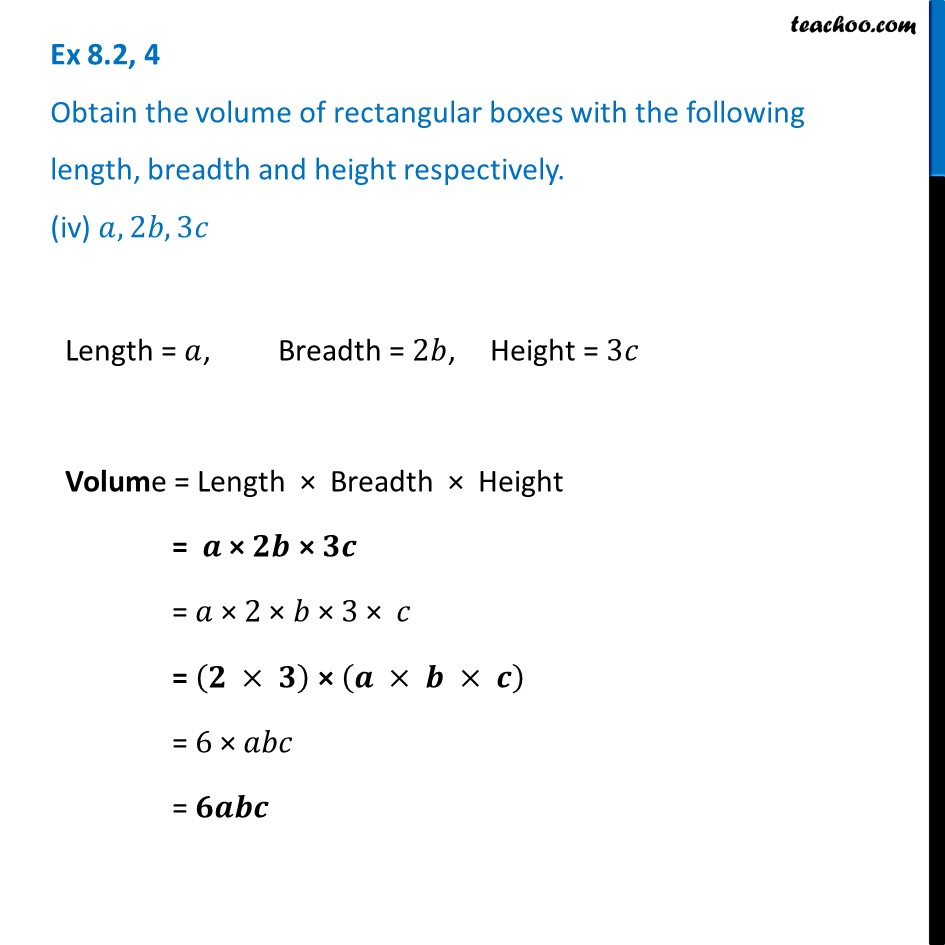Ex 8.2

Chapter 8 Class 8 Algebraic Expressions and Identities
Serial order wiseLearn in your speed, with individual attention - Teachoo Maths 1-on-1 Class

### Transcript

Ex 8.2, 4 Obtain the volume of rectangular boxes with the following length, breadth and height respectively. (iv) 𝑎, 2𝑏, 3𝑐Length = 𝑎, Breadth = 2𝑏, Height = 3𝑐 Volume = Length × Breadth × Height = 𝒂 × 𝟐𝒃 × 𝟑𝒄 = 𝑎 × 2 × 𝑏 × 3 × 𝑐 = (𝟐 × 𝟑) × (𝒂 × 𝒃 × 𝒄) = 6 × 𝑎𝑏𝑐 = 𝟔𝒂𝒃𝒄